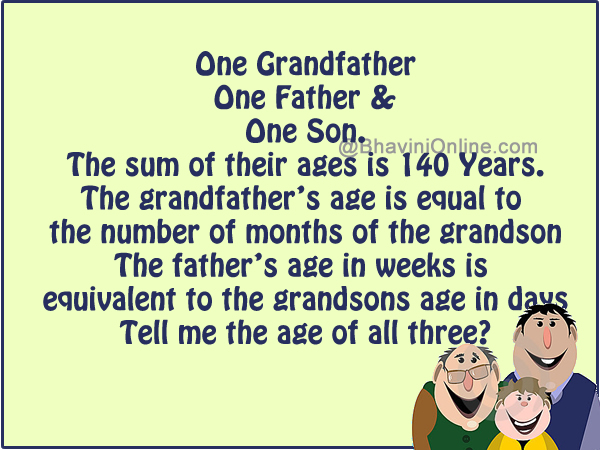# WhatsApp Riddle: What are the ages of Grandfather, Father and Son?

Challenge your brains with this WhatsApp Riddle.

Challenging questions for you.

Total Marks 100

• One Grandfather
• One Father &
• One Son.

The sum of their ages is 140 Years.

The grandfather’s age is equal to the number of months of the grandson.

The father’s age in weeks is equivalent to the grandsons age in days

Tell me the age of all three?Best Of Luck.

Let’s see who is the King of Math’s in this Group?

I had received this riddle on WhatsApp actually. Here is the original Hindi Paheli or riddle;

आपके लिये चुनौतीपूर्ण सवाल

२४ घंटे में उत्तर दें।

Total Marks 100

एक दादा

एक बाप

एक पोता

तीनो की कुल उम्र 140 Years है।

पोता जितना महीना का

दादा उतना साल का

पोता जितना दिन का

बाप उतना सप्ताह का

तो बताओ कौन कितने कितने साल का है ?

Best Of Luck

देखते हे अपने Group में Maths का बादशाह कौन..?

So were you able to solve the riddle? Leave your answers in the comment section below.

The age of all three is 140 Years.

So, x + y + z = 140 years

The age of Grandson in number of months is equal to age of Grandfather in years.

So, 12*x = z (multiplied by 12 to convert year to month)

The age of Grandson in number of days is equal to age of father in weeks.

So,   365 * x = (365 * y) / 7

Which becomes => 7x = y

So, replacing value of y and z in original equation:

x + 7x + 12x = 140

20x = 140

I.e. x = GS = 7 years

y = F = 7*7 = 49 years

z = GF = 12*7 = 84 years

You can verify this by adding them up;

7 + 49 + 84 = 140 years.

Now you got full 100 marks.